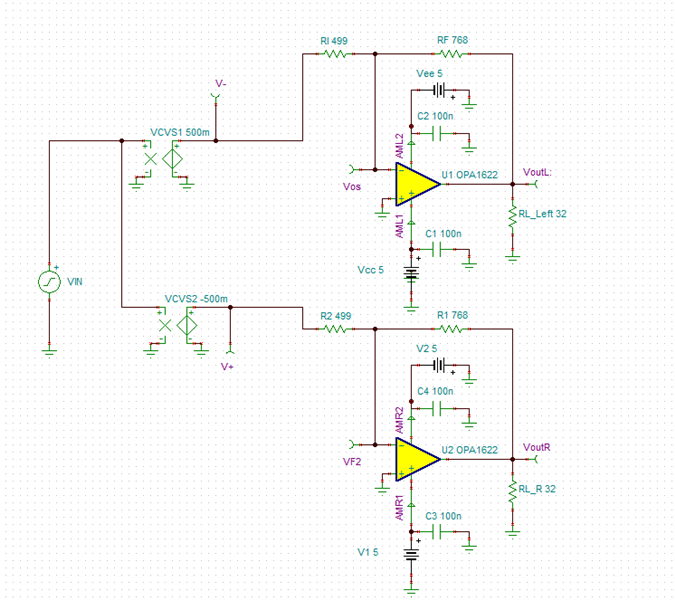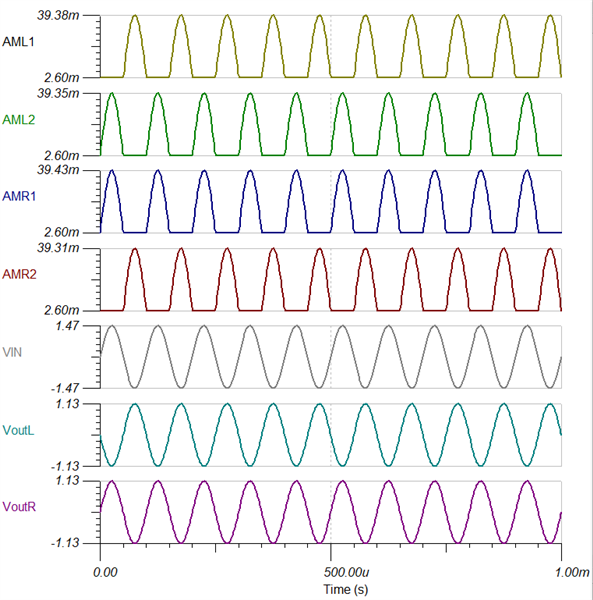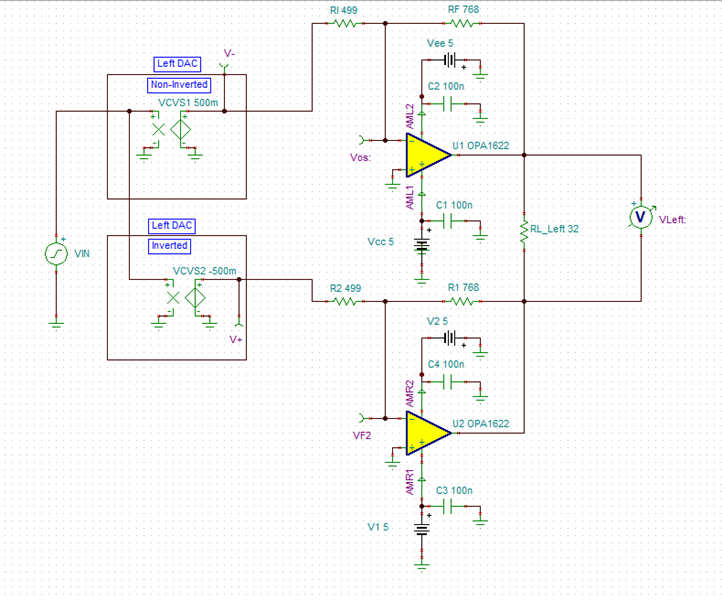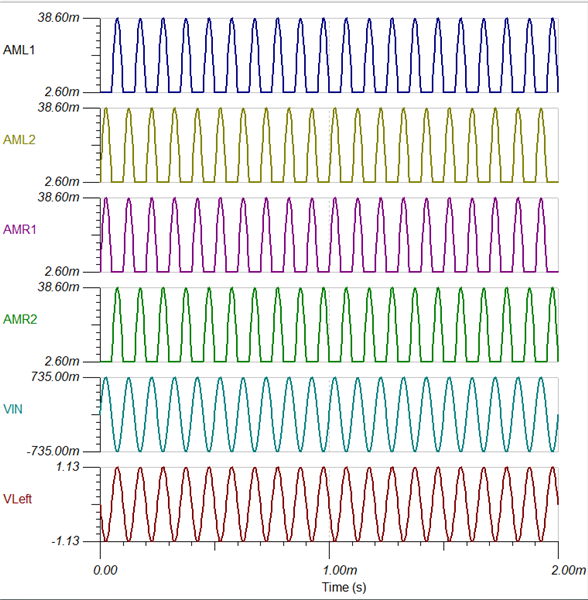If you have a related question, please click the "Ask a related question" button in the top right corner. The newly created question will be automatically linked to this question.

# OPA1622: Opa1622

Part Number: OPA1622

Hi Team,

My customer uses opa1622 for I / V and differential output stages (driving 32 ohm headphones with 40mW  per channel). The power supply voltage of opa1622 is + / - 5V. How much current does this part of the circuit need?

Thanks and regards

• Eric,

It depends on how the differential stages are setup. I have put together two topologies in Tina (attached). The first topology uses a differential output with 32 ohms at each output, one for left and one for right channels. The second topology uses one 32 ohm load between a differential output for the left channel and then a duplicate circuit for the right channel.

For 40mW per channel on 32 ohms, I am back calculating an output voltage of 1.13Vp or 2.26Vpp. Let me know if my assumption here is correct from what the customer has provided.

Topology 1:This circuit uses less current.Below you can see that the Iq of the device is 2.6mA and the current for the output signal will consume 36.78mA additional current per output. This will be roughly 78.7mA total.Topology 2: This circuit would be doubled. What is shown is just the left channel. The right channel would be a duplicate.For the left channel the total current between the two amps plus load currents will be 77.2mA. The right channel circuit would consume the same for a total of 154.4mA.